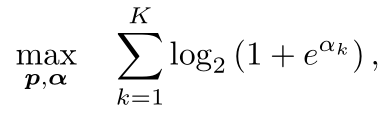# I want to ask if the expression is convex?I have a divergence with others in this problem.Thanks for help

\log(1+e^x) is a convex function. Therefore, if your objective would be min instead of max, it could appear in a convex problem. https://docs.mosek.com/modeling-cookbook/expo.html#softplus-function

so this optimized funciton is concave,right?

The function is convex. Just writing max in front of it does not suddenly make it concave.

If you want your convex optimization problem to have an objective starting with “max” then the function in the objective should be concave.